# The LIFETEST Procedure

### PROC LIFETEST Statement

PROC LIFETEST <options> ;

The PROC LIFETEST statement invokes the LIFETEST procedure. Optionally, this statement identifies an input and an OUTSURV= data set, and specifies the computation details of the survivor function estimation. Table 52.1 summarizes the options available in the PROC LIFETEST statement. These options are described in alphabetic order. If no options are requested, PROC LIFETEST computes and displays the product-limit estimate of the survivor function; and if ODS Graphics is enabled, a plot of the estimated survivor function is also displayed.

Table 52.1: Options Available in the PROC LIFETEST Statement

Option

Description

Input and Output Data Sets

Specifies the input SAS data set

Names an output data set to contain survival estimates and confidence limits

Names an output data set to contain rank test statistics for association of survival time with covariates

Nonparametric Estimation

Specifies interval endpoints for life-table estimates

Specifies the method to compute survivor function

Specifies the number of intervals for life-table estimates

Specifies the width of intervals for life-table estimates

Confidence Limits for Survivorship

Sets the confidence level for interval estimation estimates

Specifies the maximum time for confidence band

Specifies the minimum time for confidence band

Specifies the type of confidence band in the OUTSURV= data set

Specifies the transformation applied to the survivor function to obtain confidence limits

Line Printer Plots

Defines the characters used for line printer plot axes

Specifies that plots be produced by a line printer

Specifies the maximum time value for plotting

Suppresses the plot of censored observations

Specifies the plots to display

ODS Graphics

Specifies the maximum time value for plotting

Specifies plots to display

Specifies an Annotate data set that adds features to plots

Defines the symbol used for censored observations in plots

Specifies the string that appears in the description field of the PROC GREPLAY master menu for the plots

EVENTSYMBOL=

Specifies the symbol used for event observations in plots

Specifies the graphics catalog name for saving graphics output

Specifies an input data set that contains variables for local annotation

Specifies the maximum time value for plotting

Specifies the plots to display

Control Output

Adds the number of subjects at risk to the survival estimate table

Suppresses the display of printed output

Suppresses the display of survival function estimates

Displays only the estimate for the smallest time in each interval

Suppresses the Number Left column in the survival estimate table

Specifies a list of time points to display the survival estimate

Specifies that only INTERVAL= or TIMELIST= observations be listed in the OUTSURV= data set

Miscellaneous

Sets the confidence level for survival time quartiles

Allows missing values to be a stratum level

SINGULAR=

Sets the tolerance for testing singularity of covariance matrix of rank statistics

Outputs the standard error for the survival estimators to the OUTSURV= data set

Specifies the time limit used to estimate the mean survival time and its standard error

The PLOTS= option in the PROC LIFETEST statement specifies the plots to display. You can select one of the following three types of graphics in PROC LIFETEST: line printer, traditional, and ODS. If you specify the LINEPRINTER option, line printer plots are produced; otherwise traditional graphics are produced if ODS Graphics is not enabled, or ODS Graphics plots are produced if the ODS Graphics is enabled.

For more information about enabling and disabling ODS Graphics, see the section Enabling and Disabling ODS Graphics in Chapter 21: Statistical Graphics Using ODS.

Table 52.2 shows whether graphics are produced, and the type of graphics, for all possible combinations:

Table 52.2: Ways of Displaying Graphics

ODS Graphics

PLOTS= Option

LINEPRINTER Option

Graphics Results

Disabled

No

No

No graphics

Disabled

No

Yes

No graphics

Disabled

Yes

No

Disabled

Yes

Yes

Line printer plot

Enabled

No

No

ODS Graphics survival plot

Enabled

No

Yes

No graphics

Enabled

Yes

No

ODS Graphics

Enabled

Yes

Yes

Line printer plot

ODS Graphics is the preferred method of creating graphs. Many new features have been added to the ODS Graphics plots in PROC LIFETEST. For example, you can display the number of subjects at risk in a survival plot through ODS Graphics, but such a feature is not available in traditional graphics or line printer plots. The PLOTS= option syntax is documented separately for each type of graphics and is preceded by a heading that indicates the graphics type.

ALPHA=specifies the level of significancefor the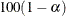% confidence intervals for the survivor, hazard, and density functions. For example, the option ALPHA=0.05 requests the 95% confidence limits for the survivor function. The default value is 0.05.

ALPHAQT=specifies the significance levelfor the% confidence intervals for the quartiles of the survival time. For example, the option ALPHAQT=0.05 requests a 95% confidence interval for the quartiles of the survival time. The default value is 0.05.

ANNOTATE=SAS-data-set
ANNO=SAS-data-set

specifies an input data set that contains appropriate variables for annotation of the traditional graphics. The ANNOTATE= option enables you to add features (for example, labels that explain extreme observations) to plots produced on graphics devices. The ANNOTATE= option cannot be used if the LINEPRINTER option is specified or if ODS Graphics is enabled. The data set specified must be an ANNOTATE= type data set, as described in SAS/GRAPH: Reference.

The data set specified with the ANNOTATE= option in the PROC LIFETEST statement is global in the sense that the information in this data set is displayed in every plot produced by a single invocation of PROC LIFETEST.

ATRISK

adds a column that represents the number of subjects at risk to the survival estimate table. Also added is a column that represents the number of events at each observed time. This option has no effect for the life-table method.

BANDMAXTIME=value
BANDMAX=value

specifies the maximum time for the confidence bands. The default is the largest observed event time. If the specified BANDMAX= time exceeds the largest observed event time, it is truncated to the largest observed event time.

BANDMINTIME=value
BANDMIN=value

specifies the minimum time for the confidence bands. The default is the smallest observed event time. For the equal-precision band, if the BANDMIN= value is less than the smallest observed event time, it is defaulted to the smallest observed event time.

CENSOREDSYMBOL=name | ’string’
CS=name | ’string’

specifies the symbol value for the censored observations in traditional graphics. The value, name or ’string’, is the symbol value specification allowed in SAS/GRAPH software. The default is CS=CIRCLE. If you want to omit plotting the censored observations, specify CS=NONE. The CENSOREDSYMBOL= option cannot be used if the LINEPRINTER option is specified or if ODS Graphics is enabled.

CONFBAND=keyword

specifies the confidence bands to be output to the OUTSURV= data set. Confidence bands are available for METHOD=KM, METHOD=BRESLOW, or METHOD=FH. You can use the following keywords:

ALL

outputs both the Hall-Wellner and the equal-precision confidence bands.

EP

outputs the equal-precision confidence bands.

HW

outputs the Hall-Wellner confidence bands.

CONFTYPE=keyword

specifies the transformation applied to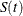to obtain the pointwise confidence intervals and the confidence bands for the survivor function in addition to the confidence intervals for the quartiles of the survival times. The following keywords can be used; the default is CONFTYPE=LOGLOG.

ASINSQRT

the arcsine-square root transformation,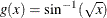LOGLOG

the log-log transformation,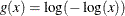This is also referred to as the log cumulative hazard transformation since it applies the logarithmic function to the cumulative hazard function. Collett (1994) and Lachin (2000) refer to it as the complementary log-log transformation.

LINEAR

the identity transformation,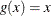LOG

the logarithmic transformation,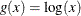LOGIT

the logit transformation,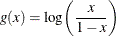DATA=SAS-data-set

names the SAS data set used by PROC LIFETEST. By default, the most recently created SAS data set is used.

DESCRIPTION='string'
DES='string'

specifies a descriptive string of up to 256 characters that appears in the Description field of the traditional graphics catalog. The description does not appear in the plots. By default, PROC LIFETEST assigns a description of the form PLOT OF vname versus hname, where vname and hname are the names of the y variable and the x variable, respectively. The DESCRIPTION= option cannot be used if the LINEPRINTER option is specified or if ODS Graphics is enabled.

EVENTSYMBOL=name | 'string'
ES=name | 'string'

specifies the symbol value for the event observations in traditional graphics. The value, name or ’string’, is the symbol value specification allowed in SAS/GRAPH software. The default is ES=NONE. The EVENTSYMBOL= option cannot be used if the LINEPRINTER option is specified or if ODS Graphics is enabled.

FORMCHAR(1,2,7,9)=’string’

defines the characters used for constructing the vertical and horizontal axes of the line printer plots. The string should be four characters. The first and second characters define the vertical and horizontal bars, respectively, which are also used in drawing the steps of the Kaplan-Meier, Breslow, or Fleming-Harrington survival curve. The third character defines the tick mark for the axes, and the fourth character defines the lower left corner of the plot. The default is FORMCHAR(1,2,7,9)='|-+-'. Any character or hexadecimal string can be used to customize the plot appearance. If you use hexadecimals, you must put an x after the closing quote. For example, to send the plot output to a printer with the IBM graphics character set (1 or 2), specify the following:

formchar(1,2,7,9)='B3C4C5C0'x


See the chapter titled The PLOT Procedure in the Base SAS Procedures Guide for further information.

GOUT=graphics-catalog

specifies the graphics catalog for saving traditional graphics output from PROC LIFETEST. The default is Work.Gseg. The GOUT= option cannot be used if the LINEPRINTER option is specified or if ODS Graphics is enabled. For more information, see the chapter titled The GREPLAY Procedure in SAS/GRAPH: Reference.

INTERVALS=values

specifies a list of interval endpoints for the life-table method. These endpoints must all be nonnegative numbers. The initial interval is assumed to start at zero whether or not zero is specified in the list. Each interval contains its lower endpoint but does not contain its upper endpoint. When this option is used with METHOD=KM, METHOD=BRESLOW, or METHOD=FH, it reduces the number of survival estimates displayed by showing only the estimates for the smallest time within each specified interval. The INTERVALS= option can be specified in any of the following ways:A list separated by blanks INTERVALS=1 3 5 7A list separated by commas INTERVALS=1,3,5,7x to y INTERVALS=1 to 7x to y BY z INTERVALS=1 to 7 by 1A combination of the above INTERVALS=1,3 to 5,7

For example, the specification

intervals=5,10 to 30 by 10


produces the set of intervals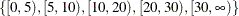LANNOTATE=SAS-data-set
LANN=SAS-data-set

specifies an input data set that contains variables for local annotation of traditional graphics. You can use the LANNOTATE= option to specify a different annotation for each BY group, in which case the BY variables must be included in the LANNOTATE= data set. The LANNOTATE= option cannot be used if the LINEPRINTER option is specified or if ODS Graphics is enabled. The data set specified must be an ANNOTATE= type data set, as described in SAS/GRAPH: Reference.

If there is no BY-group processing, the ANNOTATE= and LANNOTATE= options have the same effects.

LINEPRINTER
LS

specifies that plots are produced by a line printer instead of by a graphical device.

MAXTIME=value

specifies the maximum value of the time variable allowed on the plots so that outlying points do not determine the scale of the time axis of the plots. This option affects only the displayed plots and has no effect on any calculations.

METHOD=type

specifies the method to be used to compute the survival function estimates. Valid values for type are as follows:

BRESLOW

specifies that the Breslow estimates be computed. The Breslow estimator is the exponentiation of the negative Nelson-Aalen estimator of the cumulative hazard function.

FH

specifies that the Fleming-Harrington (FH) estimates be computed. The FH estimator is a tie-breaking modification of the Breslow estimator. If there are no tied event times, this estimator is the same as the Breslow estimator.

KM
PL

specifies that Kaplan-Meier estimates (also known as the product-limit estimates) be computed.

ACT
LIFE
LT

specifies that life-table estimates (also known as actuarial estimates) be computed.

By default, METHOD=KM.

MISSING

allows missing values for numeric variables and blank values for character variables as valid stratum levels. See the section Missing Values for details.

By default, PROC LIFETEST does not use observations with missing values for any stratum variables.

NELSON
AALEN

produces the Nelson-Aalen estimates of the cumulative hazards and the corresponding standard errors. This option is ignored if METHOD=LT is specified.

NINTERVAL=value

specifies the number of intervals used to compute the life-table estimates of the survivor function. This parameter is overridden by the WIDTH= option or the INTERVALS= option. When you specify the NINTERVAL= option, PROC LIFETEST tries to find an interval that results in round numbers for the endpoints. Consequently, the number of intervals can be different from the number requested. Use the INTERVALS= option to control the interval endpoints. The default is NINTERVAL=10.

NOCENSPLOT
NOCENS

requests that the plot of censored observations be suppressed when the LINEPRINTER and PLOTS= options are specified. This option is not needed when the life-table method is used to compute the survival estimates, because the plot of censored observations is not produced.

NOLEFT

suppresses the Number Left and Number Event columns in the survival estimate table. This option has no effect for the life-table estimate.

NOPRINT

suppresses the display of output. This option is useful when only an output data set is needed. It temporarily disables the Output Delivery System (ODS); see Chapter 20: Using the Output Delivery System, for more information.

NOTABLE

suppresses the display of survival function estimates. Only the number of censored and event times, plots, and test results is displayed.

OUTSURV=SAS-data-set
OUTS=SAS-data-set

creates an output SAS data set to contain the estimates of the survival function and corresponding confidence limits for all strata. See the section OUTSURV= Data Set for more information about the contents of the OUTSURV= data set.

OUTTEST=SAS-data-set
OUTT=SAS-data-set

creates an output SAS data set to contain the overall chi-square test statistic for association with failure time for the variables in the TEST statement, the values of the univariate rank test statistics for each variable in the TEST statement, and the estimated covariance matrix of the univariate rank test statistics. See the section OUTTEST= Data Set for more information about the contents of the OUTTEST= data set.

Line Printer PLOTS= Option

PLOTS=plot-request
PLOTS=(plot-requests)

controls the line printer plots produced. You must also specify the LINEPRINTER option to obtain line printer plots. When you specify only one plot-request, you can omit the parentheses around the plot-request. Here are some examples:

 plots=s
plots=(s ls lls)


The plot-requests include the following:

CENSORED
C

specifies a plot of censored observations. This option is available for METHOD=KM, METHOD=BRESLOW, or METHOD=FH only.

SURVIVAL
S

specifies a plot of the estimated SDF versus time.

LOGSURV
LS

specifies a plot of the negative log of the estimated SDF versus time.

LOGLOGS
LLS

specifies a plot of the log of the negative log of the estimated SDF versus the log of time.

HAZARD
H

specifies a plot of the estimated hazard function versus time (life-table method only).

PDF
P

specifies a plot of the estimated probability density function versus time (life-table method only).

ODS Graphics PLOTS= Option

PLOTS<(global-plot-option)> = plot-request <(options)>
PLOTS<(global-plot-option)> = (plot-request <(options)> <…plot-request <(options)> >)

controls the plots produced using ODS Graphics. When you specify only one plot-request, you can omit the parentheses around the plot-request. Here are some examples:

plots=none
plots=(survival(atrisk=100 to 350 by 50) logsurv)
plots(only)=hazard


ODS Graphics must be enabled before plots can be requested. For example:

ods graphics on;

proc lifetest plots=survival(atrisk);
time T*Status(0);
run;

ods graphics off;


For more information about enabling and disabling ODS Graphics, see the section Enabling and Disabling ODS Graphics in Chapter 21: Statistical Graphics Using ODS.

If ODS Graphics is enabled but you do not specify the PLOTS= option, then PROC LIFETEST produces a plot of the estimated survivor functions by default.

The only global-plot-option follows:

ONLY

specifies that only the specified plots in the list be produced; otherwise, the default survivor function plot is also displayed.

The plot-requests and plot-request options include the following.

ALL

produces all appropriate plots. For METHOD=KM, METHOD=BRESLOW, or METHOD=FH, specifying PLOTS=ALL is equivalent to specifying PLOTS=(SURVIVAL LOGSURV LOGLOGLS HAZARD); for the life-table method, PLOTS=ALL is equivalent to specifying PLOTS=(SURVIVAL LOGSURV LOGLOGS DENSITY HAZARD).

HAZARD <(hazard-options)>
H <hazard-options>

plots the estimated hazard functions. Kernel-smoothed estimates are produced for METHOD=KM, METHOD=BRESLOW, or METHOD=FH. You can specify the following hazard-options, but only the CL option can be used for the life-table method:

BANDWIDTH=bandwidth-option
BW=bandwidth-option

specifies what bandwidth is chosen for the kernel-smoothing and how it is chosen. You can specify one of the following bandwidth-options.

value

sets the bandwidth to the given value.

numeric-list

selects the bandwidth from the given numeric-list that minimizes the mean integrated squared error.

RANGE(lower,upper)

selects the bandwidth from the interval (lower, upper) that minimizes the mean integrated squared error. PROC LIFETEST uses the golden section search algorithm to find the minimum. If there is more than one local minimum in the interval, there is no guarantee that the local minimum found is also the global minimum.

See the section Optimal Bandwidth for details about the mean integrated squared error. If the BANDWIDTH= option is not specified, the default is BANDWIDTH= RANGE(0.2b,20b), where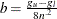,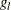and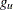are the values of the GRIDL= and GRIDU= options, respectively, and n is the total number of noncensored observations.

GRIDL=number

specifies the lower grid limit for the kernel-smoothed estimate. The default value is the time origin.

GRIDU=number

specifies the upper grid limit for the kernel-smoothed estimate. The default value equals the maximum event time.

KERNEL=kernel-option

specifies the kernel used. The choices are as follows:

BIWEIGHT
BW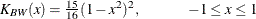EPANECHNIKOV
E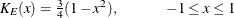UNIFORM
U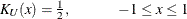The default is KERNEL=EPANECHNIKOV.

NMINGRID=number

specifies the number of grid points in determining the mean integrated square error (MISE). The default value is 51.

NGRID=number

specifies the number of grid points. The default is 101.

CL

displays the pointwise confidence limits for the smoothed hazard.

LOGLOGS
LLS

plots the log of negative log of estimated survivor functions versus the log of time.

LOGSURV
LS

plots the negative log of estimated survivor functions versus time.

NONE

suppresses all plots.

PDF <(CL)>
P <(CL)>

plots the estimated probability density functions (life-table method only). Pointwise confidence limits are displayed optionally by specifying the CL option.

SURVIVAL <(survival-options)>
S <(survival-options)>

plots the estimated survivor functions. Censored times are plotted as a plus sign on the Kaplan-Meier, Breslow, or Fleming-Harrington survival curves unless the NOCENSOR option is specified. You can customize the display by using the following survival-options. If these options are not sufficient for your purposes, you can customize the survival plot by modifying its graphical template (see the section Modifying the ODS Template for Survival Plots for more information).

ATRISK <(options)> <=number-list>

displays the numbers of subjects at risk at the given times. The following options can be specified:

ATRISKTICK
ATRISKLABEL

shows the time values at which the numbers of subjects at risk are displayed.

MAXLEN=n

specifies the number of characters n that are allowed for displaying the stratum labels. If n is greater than or equal to the maximum length of the stratum labels, the stratum labels are used in the at-risk display; otherwise, the stratum numbers are used. The default is MAXLEN=12.

OUTSIDE<(p)>

specifies that the at-risk table be drawn outside the plot area. PROC LIFETEST uses an ODS graphical template with a two-row lattice layout. The upper cell displays the survival plot, and the bottom cell displays the at-risk table. You can specify an optional number p that represents the fractional proportion of the at-risk table height relative to the overall grid height. By default, p is set to 0.035 times the number of strata, with a maximum value of 0.5.

The number-list identifies the times at which the numbers at risk are displayed. If the number-list is not specified, PROC LIFETEST uses the default list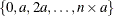, where a and n are computed by the following algorithm. Let m be the MAXTIME= value or the largest observed time if the MAXTIME= option is not specified; let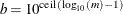, where ceil() is the ceiling function.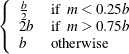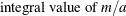CB <=keyword>

displays the confidence bands (that is, simultaneous confidence intervals) for the survivor functions. You can specify one of the following keywords. The default is CB=HW.

ALL

displays both the equal-precision and the Hall-Wellner bands.

EP

displays the equal-precision band.

HW

displays the Hall-Wellner confidence band.

CL

displays the pointwise confidence limits for the survivor functions.

FAILURE
F

changes all the displays for survivor functions to those for the failure functions. For example, if both the FAILURE and CL options are specified, the plot displays the failure curves in addition to the pointwise confidence limits for the failure functions.

NOCENSOR

suppresses the plotting of the censored times on a Kaplan-Meier, Breslow, or Fleming-Harrington survival curve.

STRATA=strata-option

specifies how to display the survival/failure curves for multiple strata. This option has no effect if there is only one stratum. You can choose one of the following strata options:

INDIVIDUAL
UNPACK

specifies that a separate plot be displayed for each stratum.

OVERLAY

specifies that the survival/failure curves for the strata be overlaid in one plot.

PANEL

specifies that separate plots for the strata be organized into panels of two or four plots, depending on the number of strata.

The default is STRATA=OVERLAY.

TEST

displays the p-value of a homogeneity test specified in the STRATA statement. If more than one test is produced, the test is chosen in the following order: LOGRANK, WILCOXON, TARONE, PETO, MODPETO, FLEMING, and LR.

PLOTS=plot-request <(NAME=name | ’string’)>
PLOTS=(plot-request <(NAME=name | ’string’)> <, …, plot-request <(NAME=name | ’string’)> >)

controls plots produced in traditional graphics. To obtain traditional graphics, you must neither enable ODS Graphics nor specify the LINEPRINTER option. For each plot-request, you can use the NAME= option to specify a name to identify the plot. The name can be specified as a SAS name or as a quoted string of up to 256 characters. Only the first eight characters are used as the entry name in the GOUT= catalog. The plot-requests include the following:

SURVIVAL
S

plots the estimated survivor functions versus time.

LOGSURV
LS

plots the negative log of estimated survivor functions versus time.

LOGLOGS
LLS

plots the log of negative log of estimated survivor functions versus the log of time.

HAZARD
H

plots estimated hazard function versus time (life-table method only).

PDF
P

plots the estimated probability density function versus time (life-table method only).

When you specify only one plot-request, you can omit the parentheses around the plot-request. Here are some examples:

 plots=s
plots=(s(name=Surv2), h(name=Haz2))


The latter requests a plot of the estimated survivor function versus time and a plot of the estimated hazard function versus time, with Surv2 and Haz2 as their names in the GOUT= catalog, respectively.

REDUCEOUT

specifies that the OUTSURV= data set contain only those observations that are included in the INTERVALS= or TIMELIST= option. This option has no effect if the OUTSURV= option is not specified. It also has no effect if neither the INTERVALS= option nor the TIMELIST= option is specified.

SINGULAR=value

specifies the tolerance for testing singularity of the covariance matrix for the rank test statistics. The test requires that a pivot for sweeping a covariance matrix be at least this number times a norm of the matrix. The default value is 1E–12.

STDERR

specifies that the standard error of the survivor function (SDF_STDERR) be output to the OUTSURV= data set. If the life-table method is used, the standard error of the density function (PDF_STDERR) and the standard error of the hazard function (HAZ_STDERR) are also output.

TIMELIM=time-limit

specifies the time limit used in the estimation of the mean survival time and its standard error. The mean survival time can be shown to be the area under the Kaplan-Meier survival curve. However, if the largest observed time in the data is censored, the area under the survival curve is not a closed area. In such a situation, you can choose a time limit L and estimate the mean survival curve limited to a time L (Lee, 1992, pp. 72–76). This option is ignored if the largest observed time is an event time. Valid time-limit values are as follows:

EVENT
LET

specifies that the time limit L be the largest event time in the data. TIMELIM=EVENT is the default.

OBSERVED
LOT

specifies that the time limit L be the largest observed time in the data.

number

specifies that the time limit L be the given number. The number must be positive and at least as large as the largest event time in the data.

TIMELIST=number-list

specifies a list of time points at which the Kaplan-Meier estimates are displayed. The time points are listed in the column labeled Timelist. Since the Kaplan-Meier survival curve is a decreasing step function, each given time point falls in an interval that has a constant survival estimate. The event time that corresponds to the beginning of the time interval is displayed along with its survival estimate.

WIDTH=value

sets the width of the intervals used in the life-table calculation of the survival function. This parameter is overridden by the INTERVALS= option.